# Learning unsupervised embeddings for textual similarity with transformers

In this article, we look at SimCSE, a simple contrastive sentence embedding framework, which can be used to produce superior sentence embeddings, from either unlabeled or labeled data. The idea behind the unsupervised SimCSE is to simply predicts the input sentence itself, with only dropout used as noise. The same input sentence is passed to the pre-trained encoder twice and obtain two embeddings as “positive pairs”, by applying independently sampled dropout masks. Due to the dropout, both sentence embeddings will be slightly different. The distance between these two embeddings will be minimized, while the distance to embeddings of the other sentences in the same batch will be maximized. Below, you see a visualization of the approach from the related paper.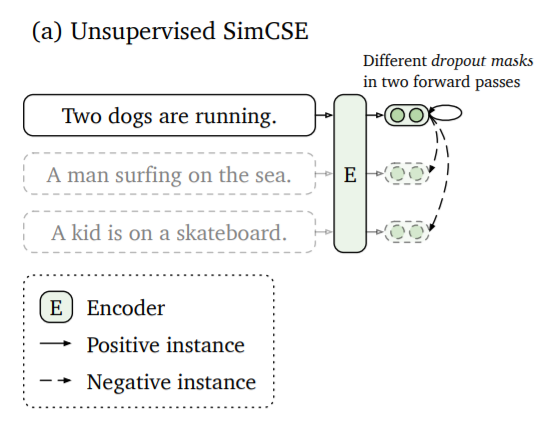## Installation and required data

I expect you, to have a standard python data science environment going already. In addition to that, you’ll need to install the following packages which we want to leverage.

pip install sentence-transformers==1.2.0
pip install umap-learn[plot]==0.5.1
pip install hdbscan==0.8.26


Once we are done with installing, we can come to the data we’re gonna use. We want to some text data, optimally with labels to evaluate our methods in some way. One interesting dataset is this dataset with Amazon product reviews. You can find it here: https://www.kaggle.com/kashnitsky/hierarchical-text-classification

The labeled training dataset contains 40000 amazon product reviews with hierarchical labels for the product category. Level 1 classes are: health personal care, toys games, beauty, pet supplies, baby products, and grocery gourmet food and we will only look at these here. So let’s load it to pandas and have a quick look.

import pandas as pd



0B000E46LYGGolden Valley Natural Buffalo JerkyA3MQDNGHDJU4MK0/03.0-1The description and photo on this product need...grocery gourmet foodmeat poultryjerky
1B000GRA6N8Westing Gameunknown0/05.0860630400This was a great book!!!! It is well thought t...toys gamesgamesunknown
2B000GRA6N8Westing Gameunknown0/05.0883008000I am a first year teacher, teaching 5th grade....toys gamesgamesunknown
3B000GRA6N8Westing Gameunknown0/05.0897696000I got the book at my bookfair at school lookin...toys gamesgamesunknown
4B00000DMDQI SPY A is For Jigsaw Puzzle 63pcunknown2/45.0911865600Hi! I'm Martine Redman and I created this puzz...toys gamespuzzlesjigsaw puzzles
reviews = data.Text.values.tolist()


## Establish a baseline and the evaluation method

What we want to do now, is figure out how well a our text representation model is doing. We try to measure the performance by

• encoding the review texts with the model in question
• run a clustering algorithm on the text representations
• use a measure to determine the agreement between the level 1 product category labels and the clusters. This will give use some understanding of how well the similarity learning approach captures the structure of the dataset.

We start of by establishing a baseline.

#### The baseline

We use vanilla pre-trained distilroberta-base from huggingface transformers and derive sentence representations by averaging the final embeddings with sentence-transformers.

from sentence_transformers import SentenceTransformer, InputExample
from sentence_transformers import models, losses
from torch.utils.data import DataLoader

model_name = 'distilroberta-base'

word_embedding_model = models.Transformer(model_name, max_seq_length=256)
pooling_model = models.Pooling(
word_embedding_model.get_word_embedding_dimension(),
pooling_mode_mean_tokens=True
)
model = SentenceTransformer(modules=[word_embedding_model, pooling_model])
base_review_encodings = model.encode(reviews)


#### Visualize the representation in two dimensions

Then we project the text representations down to two dimensions with the umap algorithm and color the dots in the scatter plot by the level 1 product category to visualize the representations.

import umap
import umap.plot

mapper = umap.UMAP().fit(base_review_encodings)

p = umap.plot.points(mapper, labels=data.Cat1)
umap.plot.show(p)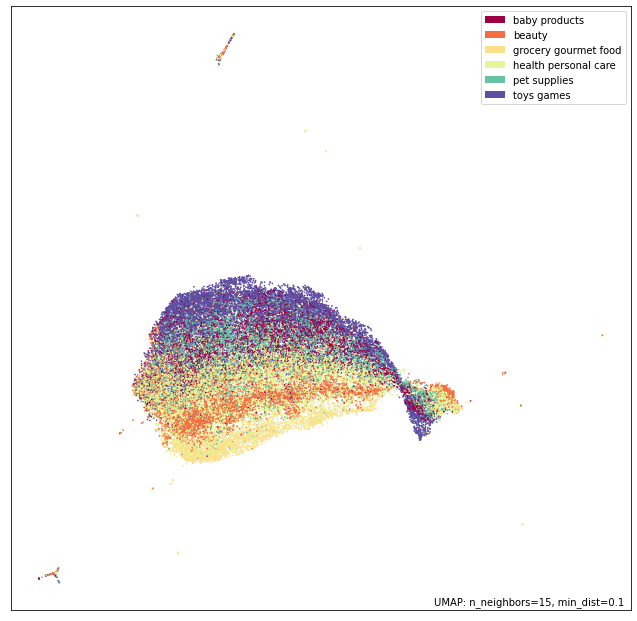We see some clear stucture in the plot, but a lot of the categories are quit mixed up. Now we try to quantify that.

#### Compute clusters and measure the agreement with the labels

By computing the k-means clusters on the text representations and measuring the agreement with the level 1 product category labels we will be able to asses the performance of the embedding method. To measure the agreement between cluster labels and category labels, we use the V-measure. This metric will give us a score between 0.0 and 1.0, where 1.0 stands for perfect agreement. Also, this metric is independent of the absolute values of the labels. So a permutation of the class or cluster label values won’t change the score value in any way. This makes it well suited for our usecase.

import hdbscan
import numpy as np
from sklearn.metrics import v_measure_score
from sklearn.cluster import KMeans

umap_embs = mapper.transform(base_review_encodings)

cluster_labels = KMeans(n_clusters=7).fit_predict(base_review_encodings)

p = umap.plot.points(mapper, labels=cluster_labels)
umap.plot.show(p)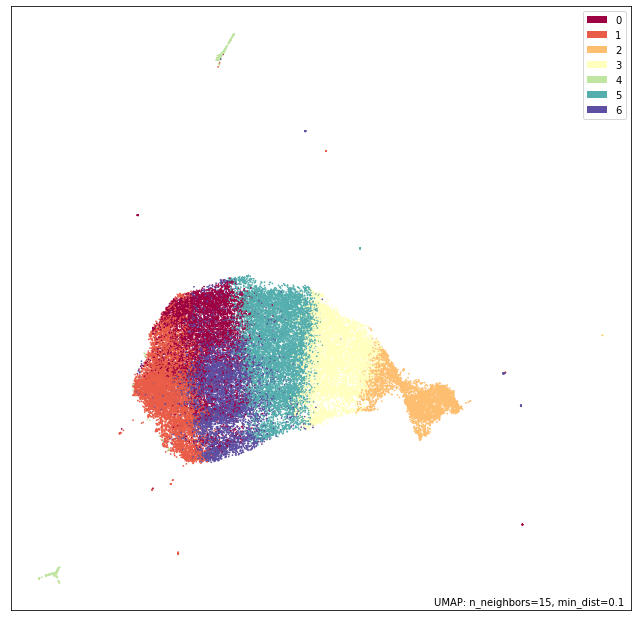Notice, that the clustering algorithm fails to capture the relevant structure compared to the labels here. Let’S calculate the V-measure:

v_score = v_measure_score(data.Cat1, cluster_labels)

0.031


We see, that the score describes our observation from above well since it is close to 0.0.

## Unsupervised text similarity with SimCSE

Now we finally come to learning a better representation in an unsupervised way.

#### Train the base model

As discussed in the beginning, we want to use the SimCSE method to train our distilroberta-base from above for the similarity task. The sentence-transformers package makes it easy to do so.

By preparing the training samples as pairs of the same text and consider them as positive pairs, we can leverage the MultipleNegativesRankingLoss. The MultipleNegativesRankingLoss is a great loss function if you only have positive pairs, like in our case. The loss expects as input a batch consisting of sentence pairs $(a_1, p_1), (a_2, p_2)…, (a_n, p_n)$ where we assume that $(a_i, p_i)$ is a positive pair and $(a_i, p_j)$ for i!=j a negative pair. For each $a_i$, it uses all other $p_j$ as negative samples, i.e., for $a_i$, we have $1$ positive example $p_i$ and $n-1$ negative examples $p_j$. It then minimizes the negative log-likelihood for softmax normalized scores.

This loss function together with the fact, that all transformer models use dropout in training by default we get the SimCSE method.

So let’s put it all together and run the training.

# Convert train sentences to positive pairs
train_data = [InputExample(texts=[text, text]) for text in reviews]

# Use the MultipleNegativesRankingLoss
train_loss = losses.MultipleNegativesRankingLoss(model)

# Call the fit method
model.fit(
epochs=2,
show_progress_bar=True
)

model.save('output/simcse-model')

Epoch:   100%|██████████| 2/2 [15:38<00:00, 469.06s/it]

Iteration:   100%|██████████| 2000/2000 [07:48<00:00, 4.27it/s]
Iteration:   100%|██████████| 2000/2000 [07:48<00:00, 4.27it/s]


#### Encode all reviews with the trained SimCSE model

Now we load the trained model back to memory and encode the review texts.

# load the trained SimCSE model
model = SentenceTransformer("./output/simcse-model/")

# encode the reviews
cse_review_encodings = model.encode(reviews)


#### Plot the umap representations

Now we can look at the umap projection of the representations again colored by the level 1 product category.

mapper = umap.UMAP().fit(cse_review_encodings)

p = umap.plot.points(mapper, labels=data.Cat1)
umap.plot.show(p)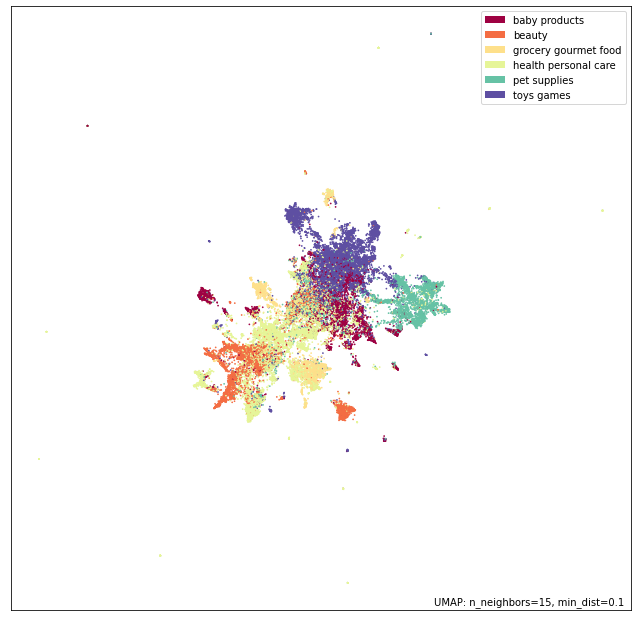It looks like the method discovered a lot of structure that seems to match the structure of the dataset well.

#### Evaluate the performance

To evaluate the performance, we repeat the steps we applied to the baseline. Instead of the k-means algorithm we use the hdbscan algorithm for clustering. This is a density based algorithm, that works well together with umap.

umap_embs = mapper.transform(cse_review_encodings)

clusterer = hdbscan.HDBSCAN(min_samples=10, min_cluster_size=1000, prediction_data=True).fit(umap_embs)
soft_clusters = hdbscan.all_points_membership_vectors(clusterer)
cluster_labels = [np.argmax(x) for x in soft_clusters]

p = umap.plot.points(mapper, labels=np.array(cluster_labels))
umap.plot.show(p)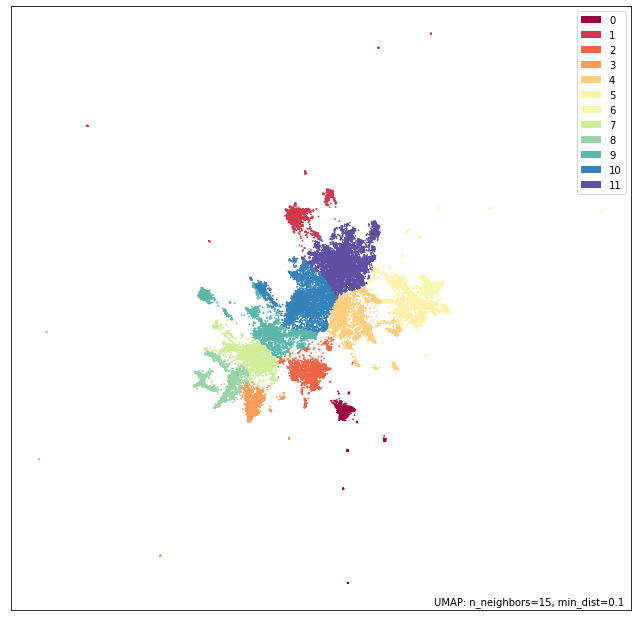This looks really nice, so let’s look if our V-measure reflects that.

v_score = v_measure_score(data.Cat1, cluster_labels)

0.39


So, thats a substantial improvement on the baseline result!

## Compare with a supervised similarity model

Finally, we close this article by comparing the similarity model we trained in an unsupervised way, to a supervised similarity model trained on several labeled sentence similarity datasets like the AllNLI dataset. We pick the paraphrase-distilroberta-base-v2 model, which has the same underlying transformer architecture as our model and is available in the sentence-transformers repository. And again, we repeat the steps from above to measure the performance of the model.

model = SentenceTransformer('paraphrase-distilroberta-base-v2')

para_review_encodings = model.encode(reviews)

mapper = umap.UMAP().fit(cse_review_encodings)

p = umap.plot.points(mapper, labels=data.Cat1)
umap.plot.show(p)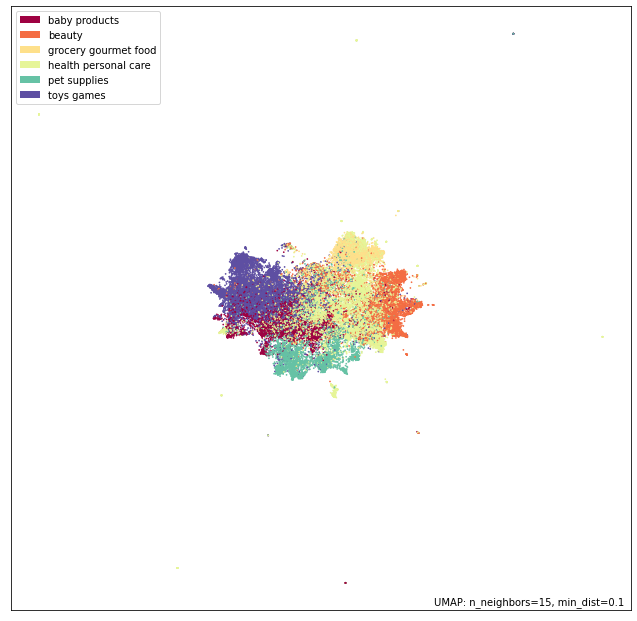umap_embs = mapper.transform(cse_review_encodings)

clusterer = hdbscan.HDBSCAN(min_samples=10, min_cluster_size=1000, prediction_data=True).fit(umap_embs)
soft_clusters = hdbscan.all_points_membership_vectors(clusterer)
cluster_labels = [np.argmax(x) for x in soft_clusters]

p = umap.plot.points(mapper, labels=np.array(cluster_labels))
umap.plot.show(p)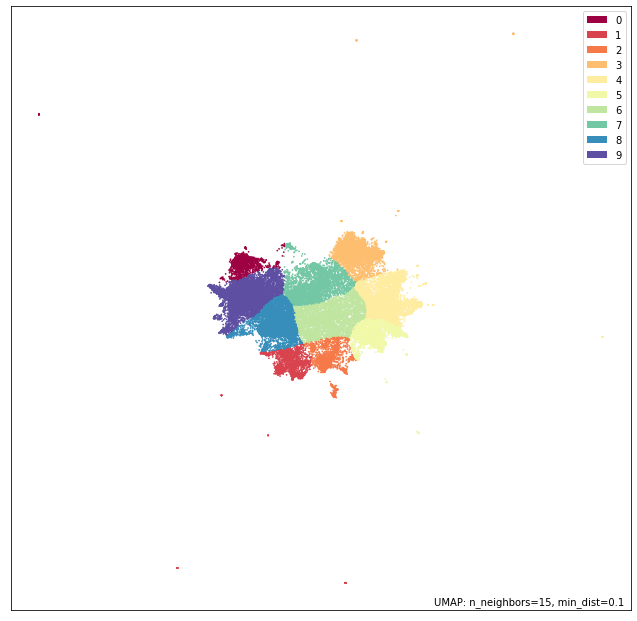The model is also able to capture quite some structure of the dataset as it looks. Let’s check the V-measure.

v_score = v_measure_score(data.Cat1, cluster_labels)

0.38


Also, much better that the baseline and on par with the unsupervised SimCSE training method.

## Conclusion

In this article, you learned, how to use the SimCSE method to learn good sentence representations in an unsupervised way. This offers a potent method for fine-tuning models for text retrieval, topic modelling and zero shot classification. Apart from that, I showed you a method to assess the performance of sentence similarity methods in a visual and quantitative way. Let me know how you applied the method and how it worked for you!

## References and futher reading